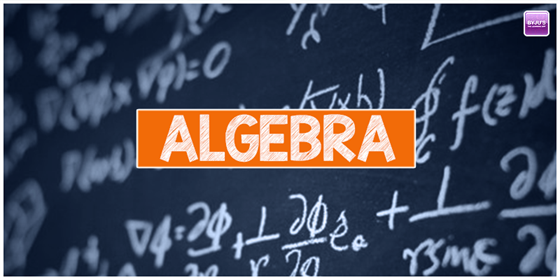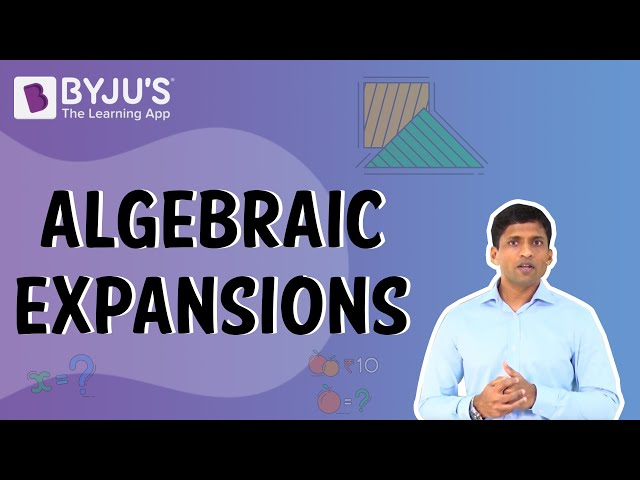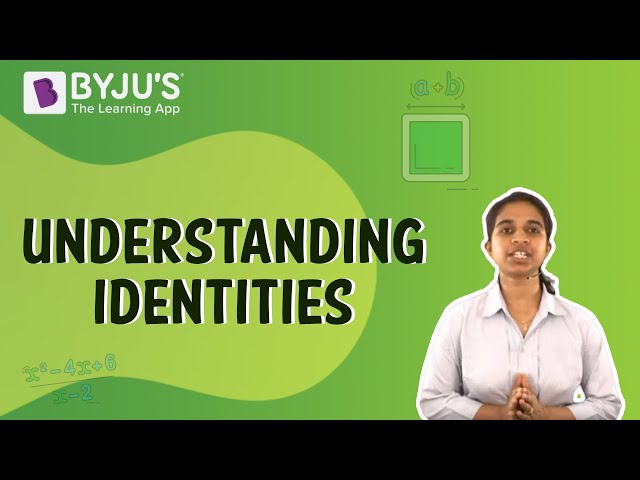Checkout JEE MAINS 2022 Question Paper Analysis : Checkout JEE MAINS 2022 Question Paper Analysis :

# Algebra

Algebra is one of the oldest branches in the history of mathematics that deals with number theory, geometry, and analysis. The definition of algebra sometimes states that the study of the mathematical symbols and the rules involves manipulating these mathematical symbols. Algebra includes almost everything right from solving elementary equations to the study of abstractions. Algebra equations are included in many chapters of Maths, which students will learn in their academics. Also, there are several formulas and identities present in algebra.

## What is Algebra?

Algebra helps solve the mathematical equations and allows to derive unknown quantities, like the bank interest, proportions, percentages. We can use the variables in the algebra to represent the unknown quantities that are coupled in such a way as to rewrite the equations.

The algebraic formulas are used in our daily lives to find the distance and volume of containers and figure out the sales prices as and when needed. Algebra is constructive in stating a mathematical equation and relationship by using letters or other symbols representing the entities. The unknown quantities in the equation can be solved through algebra.

Some of the main topics coming under algebra include Basics of algebra, exponents, simplification of algebraic expressions, polynomials, quadratic equations, etc.In BYJU’S, students will get the complete details of algebra, including its equations, terms, formulas, etc. Also, solve examples based on algebra concepts and practice worksheets to better understand the fundamentals of algebra. Algebra 1 and algebra 2 are the Maths courses included for students in their early and later stages of academics, respectively. Like, algebra 1 is the elementary algebra practised in classes 7,8 or sometimes 9, where basics of algebra are taught. But, algebra 2 is advanced algebra, which is practised at the high school level. The algebra problems will involve expressions, polynomials, the system of equations, real numbers, inequalities, etc. Learn more algebra symbols that are used in Maths.## Branches of Algebra

As it is known that,  algebra is the concept based on unknown values called variables. The important concept of algebra is equations. It follows various rules to perform arithmetic operations. The rules are used to make sense of sets of data that involve two or more variables. It is used to analyse many things around us. You will probably use the concept of algebra without realising it. Algebra is divided into different sub-branches such as elementary algebra, advanced algebra, abstract algebra, linear algebra, and commutative algebra.

### Algebra 1 or Elementary Algebra

Elementary Algebra covers the traditional topics studied in a modern elementary algebra course. Arithmetic includes numbers along with mathematical operations like +,  -,  x,  ÷. But in algebra, the numbers are often represented by the symbols and are called variables such as x, a, n, y. It also allows the common formulation of the laws of arithmetic such as, a + b = b + a and it is the first step that shows the systematic exploration of all the properties of a system of real numbers.

The concepts coming under elementary algebra include variables, evaluating expressions and equations, properties of equalities and inequalities, solving the algebraic equations and linear equations having one or two variables, etc.

### Algebra 2 or Advanced Algebra

This is the intermediate level of Algebra. This algebra has a high level of equations to solve as compared to pre-algebra. Advanced algebra will help you to go through the other parts of algebra such as:

• Equations with inequalities
• Matrices
• Solving system of linear equations
• Graphing of functions and linear equations
• Conic sections
• Polynomial Equation
• Quadratic Functions with inequalities
• Polynomials and expressions with radicals
• Sequences and series
• Rational expressions
• Trigonometry
• Discrete mathematics and probability

### Abstract Algebra

Abstract algebra is one of the divisions in algebra which discovers the truths relating to algebraic systems independent of the specific nature of some operations. These operations, in specific cases, have certain properties. Thus we can conclude some consequences of such properties. Hence this branch of mathematics called abstract algebra.

Abstract algebra deals with algebraic structures like the fields, groups, modules, rings, lattices, vector spaces, etc.

The concepts of the abstract algebra are below-

1. Sets – Sets is defined as the collection of the objects that are determined by some specific property for a set. For example – A set of all the 2×2 matrices, the set of two-dimensional vectors present in the plane and different forms of finite groups.
2. Binary Operations – When the concept of addition is conceptualized, it gives the binary operations. The concept of all the binary operations will be meaningless without a set.
3. Identity Element – The numbers 0 and 1 are conceptualized to give the idea of an identity element for a specific operation. Here, 0 is called the identity element for the addition operation, whereas 1 is called the identity element for the multiplication operation.
4. Inverse Elements – The idea of Inverse elements comes up with a negative number. For addition, we write “-a” as the inverse of “a” and for the multiplication, the inverse form is written as “a-1″.
5. Associativity – When integers are added, there is a property known as associativity in which the grouping up of numbers added does not affect the sum. Consider an example, (3 + 2) + 4 = 3 + (2 + 4)

### Linear Algebra

Linear algebra is a branch of algebra that applies to both applied as well as pure mathematics. It deals with the linear mappings between the vector spaces. It also deals with the study of planes and lines. It is the study of linear sets of equations with transformation properties. It is almost used in all areas of Mathematics. It concerns the linear equations for the linear functions with their representation in vector spaces and matrices. The important topics covered in linear algebra are as follows:

• Linear equations
• Vector Spaces
• Relations
• Matrices and matrix decomposition
• Relations and Computations

### Commutative algebra

Commutative algebra is one of the branches of algebra that studies the commutative rings and their ideals. The algebraic number theory, as well as the algebraic geometry, depends on commutative algebra. It includes rings of algebraic integers, polynomial rings, and so on. Many other mathematics areas draw upon commutative algebra in different ways, such as differential topology, invariant theory, order theory, and general topology. It has occupied a remarkable role in modern pure mathematics.

### Video Lessons

#### Algebraic Expansion#### Algebraic Identities## Solved Examples on Algebra

Example 1: Solve the equation 5x – 6 = 3x – 8.

Solution:

Given,

5x – 6 = 3x – 8

Adding 6 on both sides,

5x – 6 + 6 = 3x – 8 + 6

5x = 3x – 2

Subtract 3x from both sides,

5x – 3x = 3x – 2 – 3x

2x = -2

Dividing both sides of the equation by 2,

2x/2 = -2/2

x = -1

Example 2: Simplify:

$$\begin{array}{l}\frac{7x+5}{x-4}-\frac{6x-1}{x-3}-\frac{1}{x^2-7x+12}=1\end{array}$$

Solution:

Consider, x2 – 7x + 12

= x2 – 3x – 4x + 12

= x(x – 3) – 4(x – 3)

= (x – 4)(x – 3)

Now, from the given,

$$\begin{array}{l}\frac{7x+5}{x-4}-\frac{6x-1}{x-3}-\frac{1}{x^2-7x+12}=1\end{array}$$

Here, LCM of denominators = (x – 4)(x – 3)

Thus,

[(7x + 5)(x – 3) – (6x – 1)(x – 4) – 1]/ (x – 4)(x – 3) = 1

7x2 – 21x + 5x – 15 – (6x2 – 24x – x + 4) – 1 = (x – 4)(x – 3)

x2 + 9x – 20 = x2 – 7x + 12

9x + 7x = 12 + 20

16x = 32

x = 2

Example 3: Solve

$$\begin{array}{l}\sqrt{17x-\sqrt{x^2-5}}=7\end{array}$$

Solution:

Given,

$$\begin{array}{l}\sqrt{17x-\sqrt{x^2-5}}=7\end{array}$$

On removing the square roots of the LHS, we get;

x2 – 5 = 2401 – 1666x + 289x2

2401 – 1666x + 289x2 = x2 – 5

Adding 5 on both sides,

2401 – 1666x + 289x2 + 5 = x2 – 5 + 5

289x2 – 1666x + 2406 = x2

Subtracting x2 from sides,

289x2 – 1666x + 2406 – x2 = x2 – x2

288x2 – 1666x + 2406 = 0

$$\begin{array}{l}x=\frac{-\left(-1666\right)\pm \sqrt{\left(-1666\right)^2-4.288.2406}}{2.288}\\ x=\frac{-\left(-1666\right)\pm \:62}{576}\\ x=\frac{-\left(-1666\right)+62}{576},\:x=\frac{-\left(-1666\right)-62}{576}\end{array}$$

Therefore, x = 3, 401/144

Example 4: Solve for x:

$$\begin{array}{l}\log _2\left(x^2-6x\right)=3+\log _2\left(1-x\right)\end{array}$$

Solution:

Given,

$$\begin{array}{l}\log _2\left(x^2-6x\right)=3+\log _2\left(1-x\right)\end{array}$$

We know that, log2 base 2 = 1

so,

$$\begin{array}{l}\log _2\left(x^2-6x\right)=3\log _2 2+\log _2\left(1-x\right)\end{array}$$

⇒

$$\begin{array}{l}\log _2\left(x^2-6x\right)=\log _2 2^3+\log _2\left(1-x\right)\end{array}$$

⇒

$$\begin{array}{l}\log _2\left(x^2-6x\right)=\log _2 8+\log _2\left(1-x\right)\end{array}$$

⇒

$$\begin{array}{l}\log _2\left(x^2-6x\right)=\log _2 8\left(1-x\right)\end{array}$$

Now, by cancelling the log on both sides, we get;

(x2 – 6x) = 8(1 – x)

x2 – 6x = 8 – 8x

x2 – 6x + 8x – 8 = 0

x2 + 2x – 8 = 0

x2 + 4x – 2x – 8 = 0

x(x + 4) – 2(x + 4) = 0

(x – 2)(x + 4) = 0

Therefore, x = 2, -4

Example 5: Solve 2ex + 5 = 115

Solution:

Given,

2ex + 5 = 115

2ex = 115 – 5

2ex = 110

ex = 110/2

ex = 55

x = ln 55

### Algebra Related Articles

Stay tuned with BYJU’S – The Learning App and download the app to get all the important Maths-related articles to learn with ease.

## Frequently Asked Questions on Algebra

### What is algebra?

Algebra is a branch of mathematics that deals with solving equations and finding the values of variables. It can be used in different fields such as physics, chemistry, and economics to solve problems. Algebra is not just solving equations but also understanding the relationship between numbers, operations, and variables.

### Why should students learn algebra?

Algebra is a powerful and useful tool for problem-solving, research, and everyday life. It’s important for students to learn algebra to increase their problem-solving skills, range of understanding, and success in both maths and other subjects.

### Is algebra hard to learn?

Algebra is not that hard to learn, in fact, it can be simple and sometimes even fun. Some people say that algebra is a hard subject to learn, while others confidently say it is easy. If you think you are struggling with algebra, don’t be discouraged by what other people have told you about it; work through the problems in your textbook until you master the concepts without difficulty.

### What are the basics of algebra?

The basics of algebra are:
Addition and subtraction of algebraic expressions
Multiplications and division of algebraic expression
Solving equations
Literal equations and formulas
Applied verbal problems

### Mention the types of algebraic equations

The five main types of algebraic equations are:
Monomial or polynomial equations
Exponential equations
Trigonometric equations
Logarithmic equations
Rational equations

### What are the branches of algebra?

The branches of algebra are:
Pre-algebra
Elementary algebra
Abstract algebra
Linear algebra
Universal algebra

Test your knowledge on Algebra

1. Cecil Loving

I was doing a math example in Cube and Cuboid – Shape, Properties, Surface Area and Volume Formulas. The Example 3: Find the surface area of a cube having its sides equal to 8 cm in length.
Solution: Given length, ‘a’= 8 cm
Surface area = 6a2
= 6× 82 = 6 ×64
= 438 cm2
I have been doing this above example and I keep getting the answer of
= 384 cm2 What am I doing wrong?
6X82 = 384 6X64 = 384

• lavanya

Surface area of cube = 6a^2
= 6 x 8^2
= 6 x 8 x 8
= 384 sq.cm

2. Thanks for making this app I am so happy to learn on this app. This app makes my studies more intresting. And wonderful.

3. Splendor

I really had a great time here. I can personally say this arrangement is good